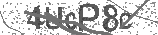﻿ The Adding by 2 Song (Math Facts) - Addition Song for Kids | Silly School Songs | Safe Videos for Kids
Welcome

# The Adding by 2 Song (Math Facts) - Addition Song for Kids | Silly School Songs

Thanks! Share it with your friends!

URL

You disliked this video. Thanks for the feedback!

Sorry, only registred users can create playlists.URLChannel: Silly School Songs
Categories: Basic Math   |   MathFind Related Videos  added
98 Views## Description

Learning to add by 2 is easy when you add this fun and catchy rhythm! For more music and information, visit http://sillyschoolsongs.com

Lyrics:

Come on, let's add by 2
Come on, let's add by 2
This is something you can totally do
Come on y'all, let's add by 2!
2 + 0 = 2
2 + 1 = 3
2 + 2 = 4
2 + 3 = 5
2 + 4 = 6
2 + 5 = 7
2 + 6 = 8
2 + 7 = 9
2 + 8 = 10
2 + 9 = 11
2 + 10 = 12

Now let's turn those numbers around

0 + 2 = 2
1 + 2 = 3
2 + 2 = 4
3 + 2 = 5
4 + 2 = 6
5 + 2 = 7
6 + 2 = 8
7 + 2 = 9
8 + 2 = 10
9 + 2 = 11
10 + 2 = 12

Now let's go up and come back down

2 + 0 = 2
2 + 1 = 3
2 + 2 = 4
2 + 3 = 5
2 + 4 = 6
2 + 5 = 7
2 + 6 = 8
2 + 7 = 9
2 + 8 = 10
2 + 9 = 11
2 + 10 = 12 Now down!
10 + 2 = 12
9 + 2 = 11
8 + 2 = 10
7 + 2 = 9
6 + 2 = 8
5 + 2 = 7
4 + 2 = 6
3 + 2 = 5
2 + 2 = 4
1 + 2 = 3
0 + 2 = 2

That's it...and now we're through!Be the first to comment